Study Guide

# The Unit Circle - Trig Equations with Non-Standard Angles

## Trig Equations with Non-Standard Angles

It's almost graduation time here at Unit Circle U. We've mastered using the unit circle with the standard angles: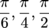and the axes. Now is a time of celebration, of reminiscing over our accomplishments and the time spent together, and really long, boring speeches.

Actually, we're skipping all of that, because we have one more thing standing between us and the tastiest graduation cake imaginable. Sometimes, we have to work with some random angle pulled off the street, instead of the angles we already know. It's so unreasonable, right?

The good thing is, from here on out we're using our calculator to help out. Take that, numbers; we have your number now. We just need to keep two things in mind:

• Make sure the calculator is set to work with radians, not degrees. Otherwise we'd have to spend all our time converting between the two, which would literally drive us up the wall. You'd need to call the fire department to get us down.
• A calculator will only give us one answer to a problem, but we know that sometimes more than one angle on the unit circle will be correct, and there are an infinite number of coterminal angles as well. We need to use our brains to finish these problems. We'd rather coast to graduation without thinking, but it was not meant to be.

### Sample Problem

Solve 5cos 2t = 2 on the interval [-π, 0].

Let's start off by getting the trig function by itself.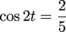Our end goal is to find t, so we need a way to lure cosine away. We had a great plan, but we couldn't afford to hire Jay-Z for an hour, and we don't think he would have gone for the bee costume anyway.

Instead, let's use the inverse cosine function, arccos or cos-1.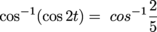2t = 1.159

STOP! Don't divide the 2 out yet. The 1.159 is actually 1.159 radians. We need to find all the other angles that equal 2t first, then do our division. To do otherwise would be…most unwise.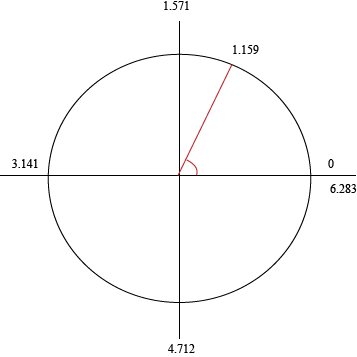This is our first set of coterminal angles. We found which quadrant they go in by relabeling the axes in decimal form, instead of using π. However, there are a second set of angles as well.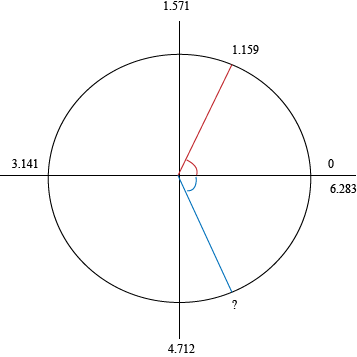We have cosine of (something) is positive when the angle is in the first or fourth quadrants. The angle will have the same corresponding acute angle of 1.159 radians, so it is 1.159 less than the x-axis. Positive angles are positively delightful, so we'll do this:

2π – 1.159 = 5.124 radians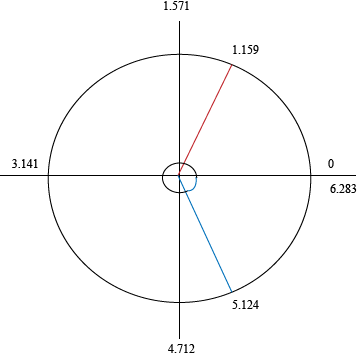Before, when we were working with the standard angles, all the possibilities were laid out for us on an already completed unit circle. Now we have to hunt for alternative angles. Luckily, we're still wearing our safari hat under our graduation cap.

Now we know all the angles equal to 2t:

2t = 1.159 + 2πn
2t = 5.124 + 2πn

From here on, things proceed as normal. We finally divide out the 2:

t = 0.5796 + πn
t = 2.562 + πn

Now we plug in values of n to find all the angles that fall in the interval [-π, 0]. Actually, we'll call it the interval [-3.141, 0] to make it easier on us.

For n = 0, t = 0.5795 and 2.562. Neither of our original angles falls in the interval. We need negative numbers. When n = -1:

t = 0.5796 – π = -2.562
t = 2.562 – π = -0.5796

These are both fine answers, real upstanding folk. For n = 2:

t = 0.5796 – 2π = -5.704
t = 2.562 – 2π = -3.712

These fall out of our interval. That means our final answer is:

t = -2.562 and -0.5796

And with that, we can finally graduate from Unit Circle U. Cake and summer vacation, here we come.